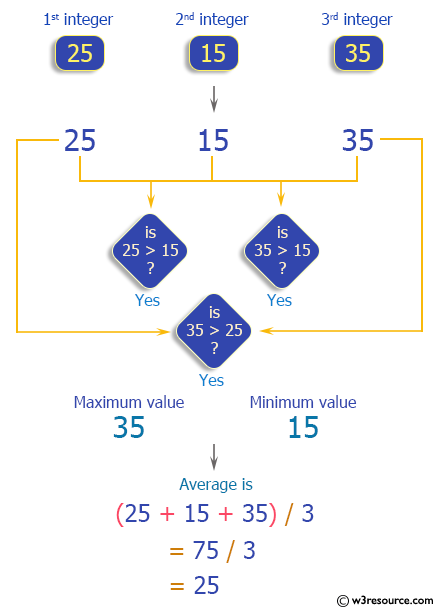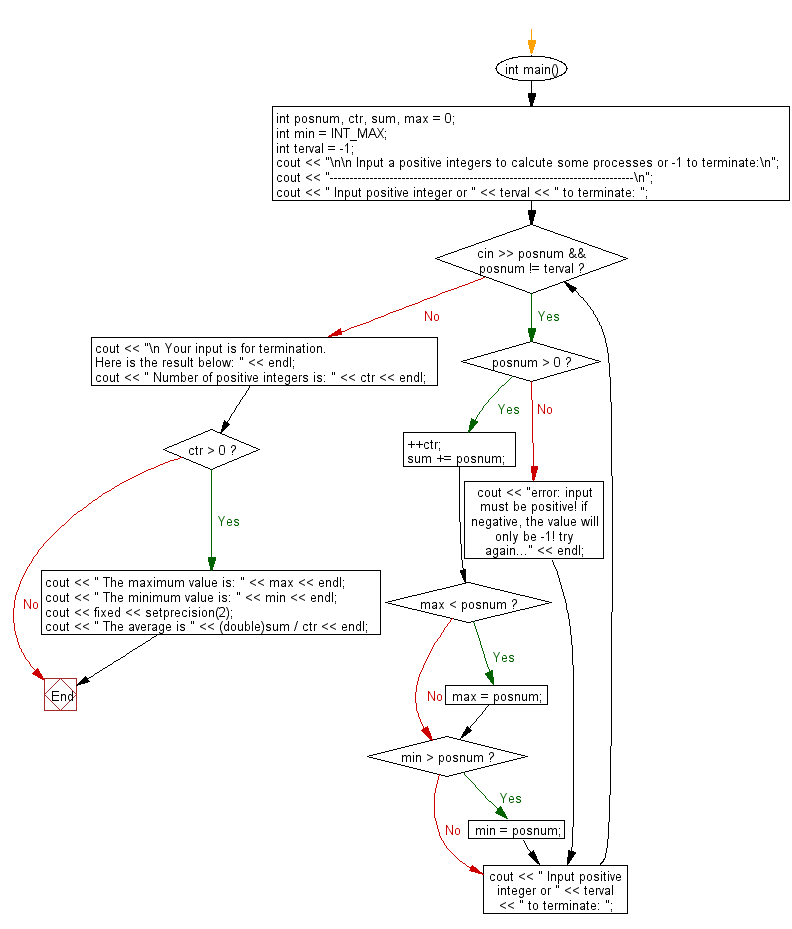﻿ C++ : Count, maximum, minimum, average of positive integers# C++ Exercises: Asked user to input positive integers to process count, maximum, minimum, and average

## C++ For Loop: Exercise-15 with Solution

Write a program in C++ to asked user to input positive integers to process count, maximum, minimum, and average or terminate the process with -1.

Pictorial Presentation:Sample Solution :-

C++ Code :

``````#include <iostream>
#include <climits>
#include <iomanip>
using namespace std;

int main()
{
int posnum, ctr, sum, max = 0;
int min = INT_MAX;
int terval = -1;
cout << "\n\n Input a positive integers to calculate some processes or -1 to terminate:\n";
cout << "----------------------------------------------------------------------------\n";
cout << " Input positive integer or " << terval << " to terminate: ";
while (cin >> posnum && posnum != terval)
{
if (posnum > 0)
{
++ctr;
sum += posnum;
if (max < posnum)
max = posnum;
if (min > posnum)
min = posnum;
}
else
{
cout << "error: input must be positive! if negative, the value will only be -1! try again..." << endl;
}
cout << " Input positive integer or " << terval << " to terminate: ";
}
cout << "\n Your input is for termination. Here is the result below: " << endl;
cout << " Number of positive integers is: " << ctr << endl;
if (ctr > 0)
{
cout << " The maximum value is: " << max << endl;
cout << " The minimum value is: " << min << endl;
cout << fixed << setprecision(2);
cout << " The average is " << (double)sum / ctr << endl;
}
}
``````

Sample Output:

``` Input a positive integers to calculate some processes or -1 to terminate:
----------------------------------------------------------------------------
Input positive integer or -1 to terminate: 25
Input positive integer or -1 to terminate: 15
Input positive integer or -1 to terminate: 35
Input positive integer or -1 to terminate: -1

Your input is for termination. Here is the result below:
Number of positive integers is: 3
The maximum value is: 35
The minimum value is: 15
The average is 25.00
```

Flowchart:C++ Code Editor:

Contribute your code and comments through Disqus.

What is the difficulty level of this exercise?

﻿

## C++ Programming: Tips of the Day

What is a smart pointer and when should I use one?

This answer is rather old, and so describes what was 'good' at the time, which was smart pointers provided by the Boost library. Since C++11, the standard library has provided sufficient smart pointers types, and so you should favour the use of std::unique_ptr, std::shared_ptr and std::weak_ptr.

There was also std::auto_ptr. It was very much like a scoped pointer, except that it also had the "special" dangerous ability to be copied - which also unexpectedly transfers ownership.

It was deprecated in C++11 and removed in C++17, so you shouldn't use it.

```std::auto_ptr<MyObject> p1 (new MyObject());
std::auto_ptr<MyObject> p2 = p1; // Copy and transfer ownership.
// p1 gets set to empty!
p2->DoSomething(); // Works.
p1->DoSomething(); // Oh oh. Hopefully raises some NULL pointer exception.
```

Ref : https://bit.ly/3mc9GHE